# Digital Electronics - Boolean Algebra and Logic Simplification - Discussion

### Discussion :: Boolean Algebra and Logic Simplification - General Questions (Q.No.1)

1.

Convert the following SOP expression to an equivalent POS expression.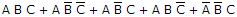[A].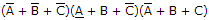[B].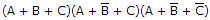[C].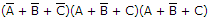[D].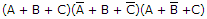Answer: Option B

Explanation:

No answer description available for this question.

 Aditya Warnulkar said: (Jan 13, 2011) Minterms are = 7, 4, 5, 6, 1. Hence corresponding maxterms will be 0, 2, 3. Therefore option B.

 Rajasekhar said: (May 18, 2011) How to find maxterms?

 Ganesh Rathod said: (Jul 22, 2011) SOP is complement of POS and vice-versa. So option B is the correct answer.

 Maha said: (Sep 7, 2011) Draw a three variable k-map and plot the given SOP forms. The remaining boxes which are not filled will give the answer for POS form. But you have to inverse each variable for example A means Abar.

 Jahoor Alam said: (Sep 25, 2011) Please draw the three variable k map & give explanation.

 Mounika said: (Nov 3, 2011) If sop is complement of pos then please explain that.

 Venkatesh said: (Nov 3, 2011) Max terms:0 2 3 a b c->000:0, a bbar c->010:2, a bbar cbar->011:3.

 Vinod said: (Nov 23, 2011) SHORT CUT :NO.OF COMBINATION POSSIBLE WITH 3 VARIABLE IS(01234567) min terms of the abv eq is: 7,4,5,6,1 IN POS IS TAKING MAX TERMS :REMAINING NUMBERS IS =0,2,3 MIN TERM IS :1 IF U GET 0 IS COMPLIMENT USING METHOD IS :SOP MAX TERM IS :0 IF U GET 1 IS COMPLEMENT USING METHOD IS :POS

 Dheeraj said: (Jan 3, 2012) Ya Vinod is correct.

 Shipra said: (Jan 23, 2012) Nice answer vinod.

 Jimmy said: (Mar 7, 2012) This answer is not correct. If maxterms are 0 =not A + not B + not C 2=not A + B + not C 3=A + not B + not C Maybe it cud be A is not correct.So no answer.

 Bryx said: (Jul 6, 2012) F(A,B,C) = m7+m4+m5+m6+m1 = A'B'C' + AB'C' + AB'C + ABC' + A'B'C F'(A,B,C)= m0 + m2 + m3 If we complement F', we get F; F = (m0 + m2 + m3)' By DeMorgan's Theorem; F = (A'B'C')'(A'BC')'(A'BC)' F = (A''+B''+C'')(A''+B'+C'')(A''+B'+C') F = (A+B+C)(A+B'+C)(A+B'+C') So answer is letter B.

 Sneha said: (Aug 6, 2013) By using k-map simplification technique we can find answer.

 Vinay said: (Aug 29, 2013) You are right @Sneha. K-map is best technique.

 Saba said: (Mar 24, 2015) Through the min terms is also effective.

 Priyanka said: (Aug 1, 2015) From the given sop we can find min terms they are: Min terms = 1, 4, 5, 6, 7. From the above, we can know that the sop & pos are compliments. So Max terms = 0, 2, 3. Then according to pos. The answer is B. There is no need of drawing k-map here because its take some extra time for that compared to this procedure.

 Anuj said: (Aug 29, 2015) In the addition expression of our original equation (Given one) there is no term of A bar / Not A. So, check in the options by only A bar / Not A analysis means no tern can have A bar / Not A in it. This is only possible in option B.

 Shivam said: (Nov 1, 2015) As @Priyanka said we are taking max term and according to that the answer is B but when I check I found A is answer according to the explanation.

 Amol Kool said: (Nov 16, 2015) Sop is not compliment of POS or vice versa both are similar.

 Sai Kishore said: (Nov 26, 2015) Solving using k maps in somewhat hard.

 Brian said: (Dec 1, 2015) I don't get how you did these?

 Prabu said: (Jan 18, 2016) Can any one explain it clearly?

 Gowthami Asirayya said: (Apr 28, 2016) The sum of products into the product of sum that means we have to replace in the place of * with +.

 Anuoluwapo Ishaq said: (Jun 20, 2016) I think the answer should be A + A'B'C.

 Sukriti said: (Jul 13, 2016) In the question, it is said the SOP form. Using K-map we find the position of respective boolean expression. That is 1, 4, 5, 6, 7 (these are the SOP forms i.e. 1). In SOP we substitute the 1 to A and 0 to bar A (eg. 101 = A (bar B) C. It is asked to find the POS form from this sop expression. From k-map, the rest positions which are '0' are the POS I. E 0, 2, 3. In POS we substitute the 1 to bar A and 0 to A (eg. 101 (bar A) B (bar C). As it is asked POS so we have to write in POS form. So 0, 2, 3 in POS form will be like the option B. ****It would be better if it has some option to attach a photo so that a photo of K-map can be attached.

 Dhuha said: (Jul 25, 2016) How to convert SOP to POS?

 Cal said: (Aug 4, 2016) Yeah, Aditya Warnulkar is correct. That's a short cut to getting your SOP from a POS expression instead of going by the truth table.

 Emmanuel Afriyie Owusu said: (Mar 10, 2017) When you find the maxterms corresponding to the question you will obtain ( 0,1,2,3,6) and when you do same for the possible answers. A. (7,5,4) B. (0,2,3) C. (0,2,2) D. (0,5,2) The answer is B because it's the only one that satisfies the SOP values of the question.

 Meghna said: (Apr 2, 2017) According to POS and SOP, the Correct answer is C.

 Priya said: (Sep 15, 2017) How to find maxterms?

 Imam said: (Nov 25, 2017) (Sop=pos) is this correct?

 Suneetha said: (Oct 5, 2018) Thanks for your answer @Vinod.

 Pacheko said: (Dec 10, 2018) The correct answer is A + A'B'C.

 Kiran Sharma said: (Oct 22, 2019) (A+B+C').(A+B'+C').(A+B'C)

 Av Reddy said: (Feb 11, 2020) How can I get an answer, I didn't understand.

 Joseph said: (Mar 3, 2021) How did you get an answer?I didn't understand. Anyone make it clear.

#### Post your comments here:

Name *:

Email   : (optional)

» Your comments will be displayed only after manual approval.

#### Current Affairs 2021

Interview Questions and Answers